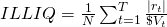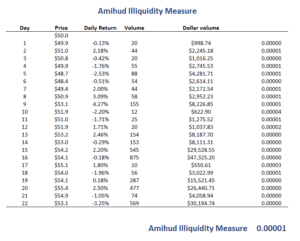# Amihud Illiquidity measure

The Amihud illiquidity measure is the most commonly used proxy of stock liquidity in the finance literature. Another popular measure which is used mainly by investors is the Average Daily Volume (ADV). The Amihud measure is mainly used to calculate the degree of illiquidity of stocks.

The measure was proposed by Yakov Amihud in 2002. The Amihud Illiquidity measure is widely used. That’s because it can easily be implemented using an Excel spreadsheet or any other software. Another advantage is that it only requires a limited amount of data.

On this page, we discuss the data needed to construct the measure, discuss the Amihud measure formula, and finally provide an Excel spreadsheet that implements the Amihud measure in Excel.

## Amihud Illiquidity Measure Definition

The fact that stocks with a high Amihud measure generate higher returns is explained as follows. These funds tend to be more illiquid, and thus investors who buy such stocks can be expected to incur higher transaction costs and market impact when they want to sell the securities. Thus, they are more exposed to illiquidity risk.

It is important to stress that the Amihud measure captures the illiquidity of a stock. Thus, if we want to know measure the liquidity, we should actually take the reciprocal of the Amihud measure. Doing so, we obtain the Amihud liquidity ratio. Next, let’s turn to the formula.

## Amihud Illiquidity Measure Formula

The Amihud formula is defined as followswhere T is the number of days, \$V is the dollar volume on day t, and rt is the return on day t. Thus, to calculate the Amihud ratio, we only need the daily dollar volume and the price changes (i.e. daily returns). These data can easily be retrieved online for most stocks.

## Amihud Illiquidity Measure Example

Finally, we implement a simple example of the Amihud measure using an Excel spreadsheet. The following figure illustrates the results that we obtain when we implement the Amihud ratio using an Excel spreadsheet. In this particular case, we calculate the ILLIQ every month using the daily returns and dollar volume for every day.Notice that the Amihud measure itself has little interpretation. That is because the measure is most commonly used to compare securities or to look at the evolution of a stock’s liquidity.

## Summary

We discussed the Amihud measure. This is the most popular measure of stock illiquidity used in the finance industry, together with average daily volume (ADV).

### Liquidity calculator

An Amihud illiquidity measure Excel implementation can be downloaded here: Amihud Illiquidity Measure